## Mathematics used in the Amorphous Maze program

Amorphous maze creation algorithm is based on starting from an outermost wall and extending the wall by a small segment at a time in a manner that avoids walls from crossing over each other. Each time a line segment is successfully extended, it is added to a global repository of line segments to test against. When the extending operation comes to a dead end, a different coordinate is randomly chosen from the repository as a starting point of a new branch.

### Distance between two pointsThere are various sections within my program that need to compute the distance between two points.  If P1=(x1,y1) and P2=(x2,y2), then  by the Pythagorean theorem, distance between two points P1 and P2 is: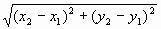### In-Out function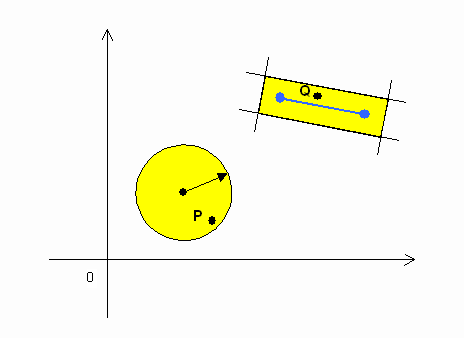Sometimes, we need to calculate if a given point lies within a circle or a rectangle.  We can test if a certain point P lies inside or outside a circle by calculating the distance between P and the center of the circle using the aforementioned distance formula. As for the rectangle, we can tell if a certain point Q lies inside or outside of a bounded rectangle by conducting four tests that tell on which side of a line a point lies. Which leads to the next topic of:

### Inner Product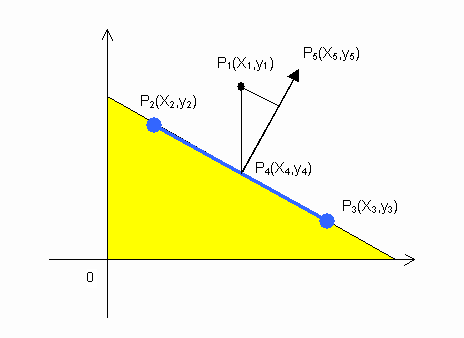There are several ways to determine on which side of a line segment P2P3 a point P1 lies. One way is to find the equation of the line y=ax+b that passes through the two points and test if y<ax+b, but this runs into difficulty when the line is perfectly vertical. A better way is to find the normal vector P4P5 emanating from the center point P4 of the line segment and compute the inner product of vectors P1P4 and P4P5.Iff the inner product is positive, P1 lies on the white side of the line segment and iff negative, the yellow side in the picture above.

### Rotation formulaSometimes we need to rotate a point.  The formula for rotating a point P1 theta radians counter clockwise about the origin, resulting in P2, is: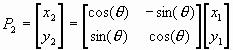### Parametric representation of a line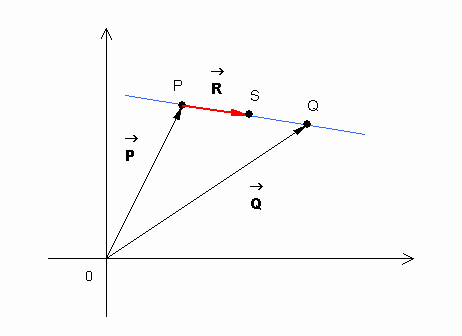If we want to test if two line segments intersect, one method is to write the equations of the lines in the format of y=a1x+b1, y=a2x+b2 and solve for (x,y). The problem with this approach is that it cannot handle perfectly vertical lines. To overcome this, we introduce a third scalar variable k. Consider a single line segment with end points P and Q.  Vector originating at point P and terminating at point Q is ( QP ).  Therefore, a vector R parallel to this line is

R = k( QP )

where k is an arbitrary scalar constant.  Therefore, all points on the line can be expressed as

S = P+k( QP )

This form is called the parametric representation of a line.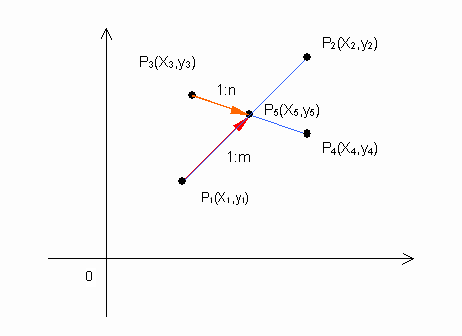### Test for Intersection of two line segments

By expressing the two line segments parametrically, it is relatively simple to determine if line segments 1 and 2 intersect.  Let

• Line segment 1: (x1,y1)(x2,y2)
• Line segment 2: (x3,y3)(x4,y4)
• Intersection, if exists, (x5,y5)

Using the parametric representation of lines

• P5=P1+m(P2-P1)
• P5=P3+n(P4-P3)

Where m and n are the amount by which P5 is into each line segments.  I.e., m is the distance of P1 to P5, normalized with respect to length of line segment 1 and n is the distance of P3 to P5, normalized with respect to length of line segment 2.  In the figure above, if the intersection lies to the left of P1, m becomes negative, and if the intersection lies to the right of P2, m becomes greater than one.  Solving for the intersection requires solving the following four simultaneous equations:

• x5=x1+(x2-x1)m
• y5=y1+(y2-y1)m
• x5=x3+(x4-x3)n
• y5=y3+(y4-y3)n

Eliminating x5 and y5,

• x1+(x2-x1)m=x3+(x4-x3)n
• y1+(y2-y1)m=y3+(y4-y3)n

Using Cramer's rule to solve this pair of equations for m and n,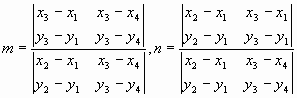We need to satisfy both 0<m<1 and 0<n<1.  Write m and n as: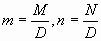where M and N are numerators whose signs are adjusted so that the denominator D is always positive.

If the lines are parallel, D, the determinant at the denominator is zero and will cause a divide-by-zero error. So rather than evaluating M/D or N/D, we take the numerators and denominators apart and test for the following four conditions:

1. 0<M
2. M<D
3. 0<N
4. N<D

The line segments 1 and 2 intersect if and only if these four conditions are met.

Last update 2011-07-31